## Unity and Phi^3

Discussion concerning the first major re-evaluation of Dewey B. Larson's Reciprocal System of theory, updated to include counterspace (Etheric spaces), projective geometry, and the non-local aspects of time/space.
ckiit
Posts: 35
Joined: Sat Dec 21, 2019 7:54 am

### Unity and Phi^3

EDIT 2020/02/26

This thread culminated into the derivation of a more precise calculation of π as shown here: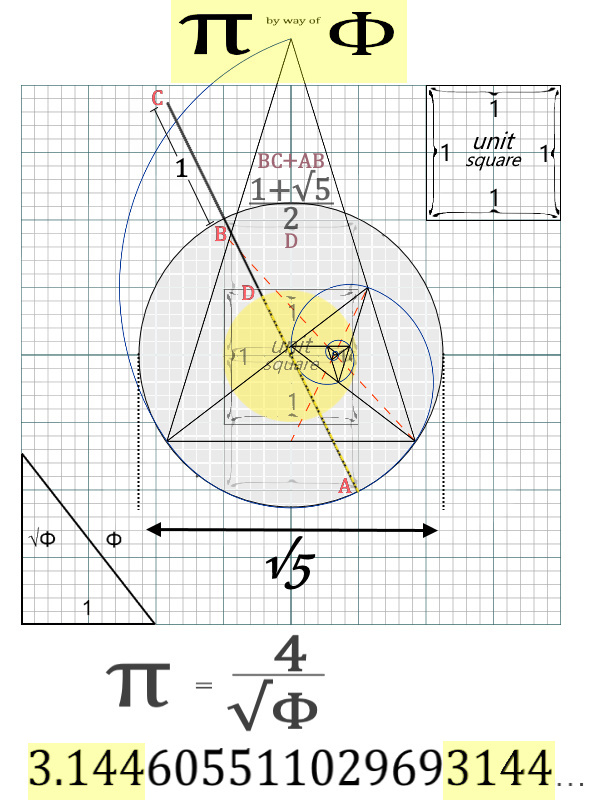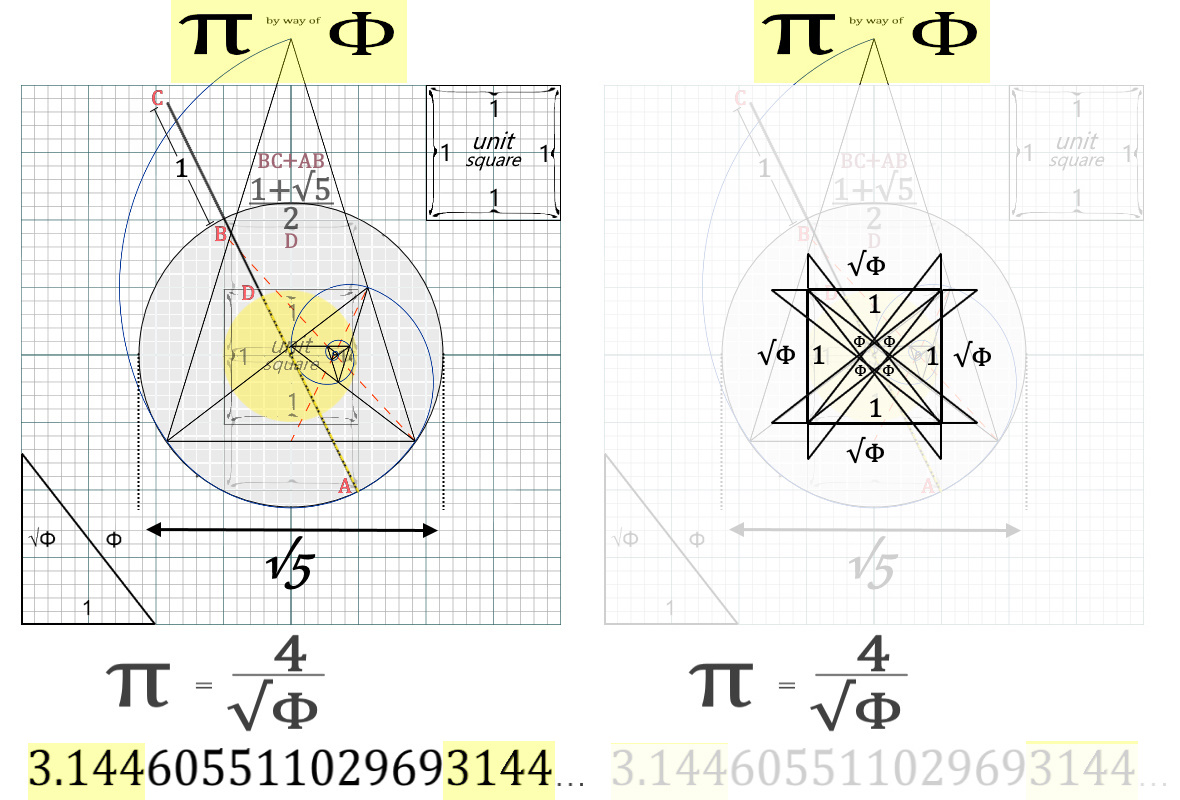Beginning with a circle whose diameter is √5,
place two unit squares inside of the circle side-by-side
(either horizontally or vertically, the latter is shown above)
and find √5 as any diagonal between two opposite corners (AB as shown)
(this diagonal thus being equal to the diameter of the circle).
Add 1 unit distance to this diagonal (√5 + 1) and find the mid-point (/2).
Note: this midpoint coincides with the circle whose diameter is 2r = 1
by way of rotating the √5 diagonal about the origin of the circle we began with,
and thus "kisses" the square four times equidistantly, thus a more precise π
can be begotten directly from Φ expressed as a ratio of Φ.
This ratio is 4/√Φ = π and/or 16/Φ = π².
and elaborated on page 3.

Concerning: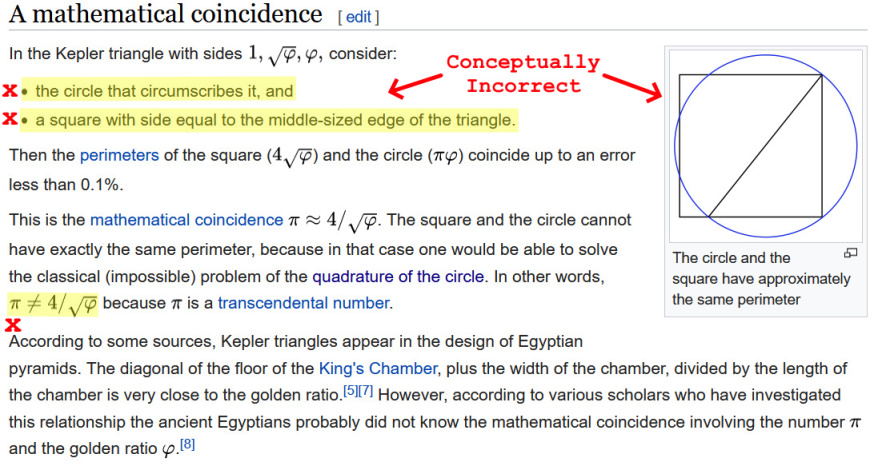https://en.wikipedia.org/wiki/Kepler_triangle

I am unsure as to the motive for the above, but the construction of the geometry
is conceptually unsound: concerning the same Kepler triangle, a circle that circumscribes it
completely ignores the imperative need for the diameter of the circle to be √5, the same to which
the Kepler triangle must apply. If the circle is not √5, there is no valid coupling and π remains 'transcendental'
however if coupled to the √5 diameter circle (as shown), the coupled relation permits a geometry
whose co-operation between Φ and π are respectively reflected in the following:

Φ solves for
f(x) = x² - x - 1
π as 4/√Φ solves for
f(x)=x4 + 16x2 - 256
thus
Φπ² = 16

graphed with an overlay of the same geometry: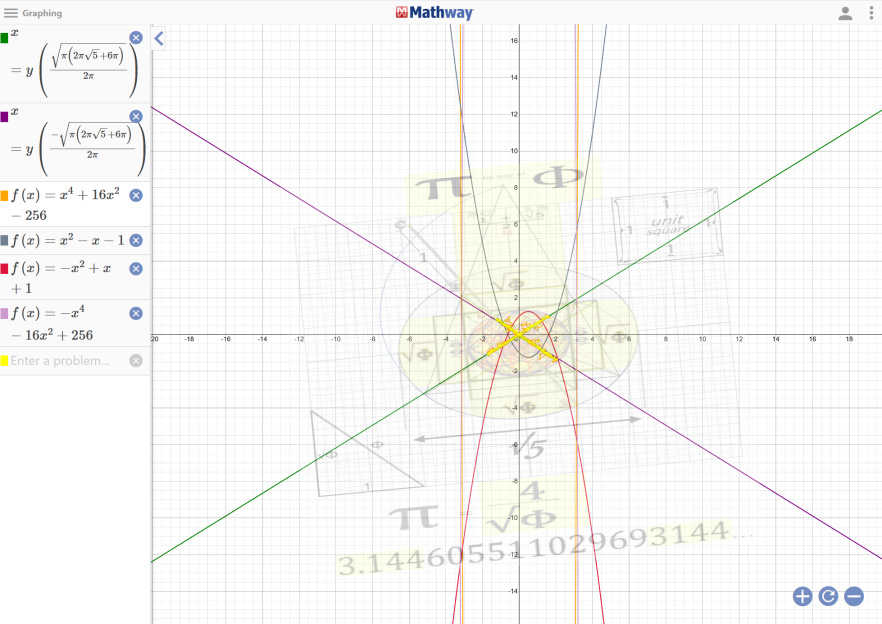__________________________________________________________________________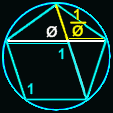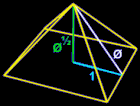This is important because it confirms the bi-rotation model (mandated by the geometry as intrinsic)
and implies a bi-orientation compliment, the properties of which can be derived inductively -
the implications of which amounts to the capacity to calculate universal roots using universal geometries
that satisfy cubed proportionality (as time and space reciprocally do).

user737
Posts: 75
Joined: Wed Oct 24, 2018 7:39 pm

### Re: Unity and Phi^3

It might be of interest to you to review the attached file for my thoughts on the matter.

Of note, it looks as though there is a mathematical corollary to the geometric concept of dimensional reduction/expansion with regard to phi

Φ = (1 + √5)/2
Attachmentsplaying_with_phi.xlsx
Infinite Rider on the Big Dogma

ckiit
Posts: 35
Joined: Sat Dec 21, 2019 7:54 am

### Re: Unity and Phi^3

user737 wrote:
Tue Jan 28, 2020 8:42 pm
It might be of interest to you to review the attached file for my thoughts on the matter.

Of note, it looks as though there is a mathematical corollary to the geometric concept of dimensional reduction/expansion with regard to phi

Φ = (1 + √5)/2
Thanks, this is great: unfortunately I can not open office files on this computer, but will take a look when possible.

What are the odds of a single identity whose +/- root solutions are +1 and +phi^3, thus scale invariant?
Would that not be an equation which describes the physical universe?

user737
Posts: 75
Joined: Wed Oct 24, 2018 7:39 pm

### Re: Unity and Phi^3

ckiit wrote:
Wed Jan 29, 2020 3:55 am
What are the odds of a single polynomial whose +/- root solutions are +1 and +phi^3, thus scale invariant?
Would that not be an equation which describes the physical universe?
3 = Φ2 + Φ = Φ2 / (Φ-1) = (Φ+1) / (Φ-1) = -Φ × Φ2= -Φ3

(1/Φ)2 + 1/Φ = +1

where Φ = (1 + √5)/2 -- the golden ratio
Infinite Rider on the Big Dogma

ckiit
Posts: 35
Joined: Sat Dec 21, 2019 7:54 am

### Re: Unity and Phi^3

user737 wrote:
Wed Jan 29, 2020 2:46 pm
ckiit wrote:
Wed Jan 29, 2020 3:55 am
What are the odds of a single polynomial whose +/- root solutions are +1 and +phi^3, thus scale invariant?
Would that not be an equation which describes the physical universe?
Φ3 = Φ2 + Φ = Φ2 / (Φ-1) = (Φ+1) / (Φ-1) = -Φ3

(1/Φ)2 + 1/Φ = 1
But (1/Φ)2 + 1/Φ
≠ 1, 4.23606797...
must equal both simultaneously.

user737
Posts: 75
Joined: Wed Oct 24, 2018 7:39 pm

### Re: Unity and Phi^3

ckiit wrote:
Wed Jan 29, 2020 3:05 pm
But (1/Φ)2 + 1/Φ
≠ 1, 4.23606797...
must equal both simultaneously.
Must be missing the "imaginary" root... missing the spin ("yin") component.
Rotational (π) component is not considered, only linear ("yang").

Must use quantum π or yin π.

yang/diagrammatic π = 3.14159...

yin/kinematic/quantum π = 4

One electric displacement is 8 natural units and one magnetic displacement is 4 natural units (as magnetic:electric is 2:1)

[(Φ2 + Φ)][(1/Φ)2 + (1/Φ)] = 1 + (1/Φ) + Φ + 1

2 + Φ + (1/Φ) = 4.236067977 (Φ3)

Also where Φ = +1 the result is 4 or π

Checking work...
Infinite Rider on the Big Dogma

ckiit
Posts: 35
Joined: Sat Dec 21, 2019 7:54 am

### Re: Unity and Phi^3

user737 wrote:
Wed Jan 29, 2020 3:15 pm
ckiit wrote:
Wed Jan 29, 2020 3:05 pm
But (1/Φ)2 + 1/Φ
≠ 1, 4.23606797...
must equal both simultaneously.
Must be missing the "imaginary" root... missing the spin ("yin") component.
Rotational (π) component is not considered, only linear ("yang").

Must use quantum π or yin π.

yang/diagrammatic π = 3.14159...

yin/kinematic/quantum π = 4

One electric displacement is 8 natural units and one magnetic displacement is 4 natural units (as magnetic:electric is 2:1)

[(Φ2 + Φ)][(1/Φ)2 + (1/Φ)] = 1 + (1/Φ) + Φ + 1

2 + Φ + (1/Φ) = 4.236067977 (Φ3)

Also where Φ = +1 the result is 4 or π

Checking work...
Both solutions are "sitting" on coefficient π2:
when setting to ^1 = pie
when setting ^2 = phi
they are thus joined.

user737
Posts: 75
Joined: Wed Oct 24, 2018 7:39 pm

### Re: Unity and Phi^3

Go ask Plato what he thinks about 1-1-Phi-1/Phi (as we have derived herein)
Infinite Rider on the Big Dogma

ckiit
Posts: 35
Joined: Sat Dec 21, 2019 7:54 am

### Re: Unity and Phi^3

user737 wrote:
Wed Jan 29, 2020 3:15 pm

yin/kinematic/quantum π = 4

One electric displacement is 8 natural units and one magnetic displacement is 4 natural units (as magnetic:electric is 2:1)

[(Φ2 + Φ)][(1/Φ)2 + (1/Φ)] = 1 + (1/Φ) + Φ + 1

2 + Φ + (1/Φ) = 4.236067977 (Φ3)

Also where Φ = +1 the result is 4 or π

Checking work...
phi of 16 = 8x2
pi of 16 = 4x4

Does the value of pi modify to 4 if Φ = +1?

ckiit
Posts: 35
Joined: Sat Dec 21, 2019 7:54 am

### Re: Unity and Phi^3

I have recovered an expression[/u] whose inverse identities are one another: 1 and Φ³

This expression (which is also a psuedo-quadratic) relates to the Reimann Hypothesis in that
it proves that all non-trivial zeros have nowhere else to go but the 1/2 line, therefor the algorithm which determines
the distribution of primes is attainable. This identity is the real value of '1' in relation to both: Φ and π
all in a single expression whose multiplicative inverse is Φ³. Therefor, Φ³/1 and 1/Φ³
is where the real number system begins/ends (ie. as like an ∞ fabric) whose "sum" is -1/12.

The reason for this strange sum relates to the numerator/denominator
progressively collapsing from bases 180→90→45→36→18→*16→*12
whose symmetric "pattern" is a consistent 9→9→9→9→9→*7→*3
ending with 12π/144π:
11/12 → 12/12 = 1/12
13/12 → 12/12 = -1/12
11/12 → (+) 1/12 → ***!1!*** ← 1/12 (-) ← 13/12
therefor the actual "sum" of -1/12 is an artifact, but this artifact is pointing to something extremely important:
because the real number system is enclosed, the identity of the psuedo-quadratic "encodes" information about the primes
such that the whole number '1' in relation to Φ and π is the "blueprint" of all possible primes. This would predict that the identity
must always remain '1' regardless of how many √ operations are performed on it.

I am very sorry to hear of Bruce's health issues - I hope his health endures to find this,
I know it will make RS2 stronger, if not a new 'theory of everything' inductively rooted
in the same language the universe itself obeys.